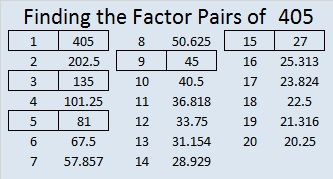# 405 and Level 3

405 times 2 equals 810, and 810 divided by 10 is 81. And guess what, 405 divided by 5 is also 81. Multiplying by .2 instead of dividing by 5 makes a nice division trick!

All of 405’s factors are listed below the puzzle.Print the puzzles or type the factors on this excel file: 12 Factors 2015-02-23

• 405 is a composite number.
• Prime factorization: 405 = 3 x 3 x 3 x 3 x 5, which can be written 405 = (3^4) x 5
• The exponents in the prime factorization are 4 and 1. Adding one to each and multiplying we get (4 + 1)(1 + 1) = 5 x 2 = 10. Therefore 405 has exactly 10 factors.
• Factors of 405: 1, 3, 5, 9, 15, 27, 45, 81, 135, 405
• Factor pairs: 405 = 1 x 405, 3 x 135, 5 x 81, 9 x 45, or 15 x 27
• Taking the factor pair with the largest square number factor, we get √405 = (√81)(√5) = 9√5 ≈ 20.1246A Logical Approach to FIND THE FACTORS: Find the column or row with two clues and find their common factor. Write the corresponding factors in the factor column (1st column) and factor row (top row).  Because this is a level three puzzle, you have now written a factor at the top of the factor column. Continue to work from the top of the factor column to the bottom, finding factors and filling in the factor column and the factor row one cell at a time as you go.This site uses Akismet to reduce spam. Learn how your comment data is processed.